Test: Frequency Domain Analysis of Control Systems- 3

# Test: Frequency Domain Analysis of Control Systems- 3

Test Description

## 20 Questions MCQ Test GATE ECE (Electronics) 2023 Mock Test Series | Test: Frequency Domain Analysis of Control Systems- 3

Test: Frequency Domain Analysis of Control Systems- 3 for Electrical Engineering (EE) 2023 is part of GATE ECE (Electronics) 2023 Mock Test Series preparation. The Test: Frequency Domain Analysis of Control Systems- 3 questions and answers have been prepared according to the Electrical Engineering (EE) exam syllabus.The Test: Frequency Domain Analysis of Control Systems- 3 MCQs are made for Electrical Engineering (EE) 2023 Exam. Find important definitions, questions, notes, meanings, examples, exercises, MCQs and online tests for Test: Frequency Domain Analysis of Control Systems- 3 below.
Solutions of Test: Frequency Domain Analysis of Control Systems- 3 questions in English are available as part of our GATE ECE (Electronics) 2023 Mock Test Series for Electrical Engineering (EE) & Test: Frequency Domain Analysis of Control Systems- 3 solutions in Hindi for GATE ECE (Electronics) 2023 Mock Test Series course. Download more important topics, notes, lectures and mock test series for Electrical Engineering (EE) Exam by signing up for free. Attempt Test: Frequency Domain Analysis of Control Systems- 3 | 20 questions in 60 minutes | Mock test for Electrical Engineering (EE) preparation | Free important questions MCQ to study GATE ECE (Electronics) 2023 Mock Test Series for Electrical Engineering (EE) Exam | Download free PDF with solutions
 1 Crore+ students have signed up on EduRev. Have you?
Test: Frequency Domain Analysis of Control Systems- 3 - Question 1

### Phase crossover frequency is defined at

Test: Frequency Domain Analysis of Control Systems- 3 - Question 2

### The Bode plot for a transfer function is shown below: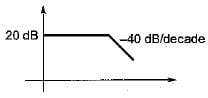The steady state error corresponding to a parabolic input is

Detailed Solution for Test: Frequency Domain Analysis of Control Systems- 3 - Question 2

Initial slope of the system = 0 dB/decade
Therefore, given system is a type-0 system.
For type-0 system, for parabolic input, steady state error is infinite since,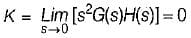Test: Frequency Domain Analysis of Control Systems- 3 - Question 3

### The frequency at which the Nyquist plot crosses the negative real axis is known as

Detailed Solution for Test: Frequency Domain Analysis of Control Systems- 3 - Question 3

At ω = ωgc, ϕ = -180°
i.e. Nyquist plot crosses the negative real axis.

Test: Frequency Domain Analysis of Control Systems- 3 - Question 4

A unity feedback system has a forward path gain of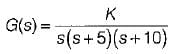The phase angle of the Nyquist plot for ω → ∞ is

Detailed Solution for Test: Frequency Domain Analysis of Control Systems- 3 - Question 4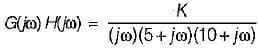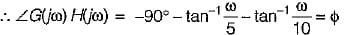At ω → ∞, ϕ = -90° - 90° - 90° = -270° = +90°

Test: Frequency Domain Analysis of Control Systems- 3 - Question 5

The open loop transfer function of a system is given as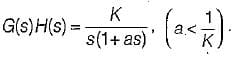The Bode plot of this system is represented as:

Detailed Solution for Test: Frequency Domain Analysis of Control Systems- 3 - Question 5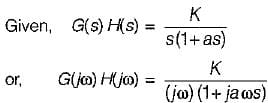= -6 dB/octave (Since Type = 1)
Corner frequency is,
= -6 dB/octave (Since Type = 1)
Corner frequency is,
It will cross 0 dB line at ω = K and K < 1/a.
So, the Bode plot will be as shown below.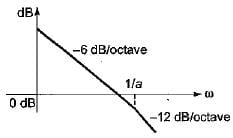Test: Frequency Domain Analysis of Control Systems- 3 - Question 6

A second order system has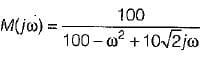It’s Mp (peak magnitude) will be approximately

Detailed Solution for Test: Frequency Domain Analysis of Control Systems- 3 - Question 6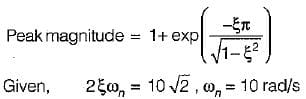∴ ξ = 1/√2
So, peak magnitude = 1 + e-π ≈ 1

Test: Frequency Domain Analysis of Control Systems- 3 - Question 7

The closed loop transfer function of a control system is given by
C(s)/R(s) = 1/(1+s)
For the input r(t) = sint, the steady state value of c(t) is equal to

Detailed Solution for Test: Frequency Domain Analysis of Control Systems- 3 - Question 7

Given,
C(s)/R(s) = 1/(1+s)
Thus,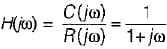Here,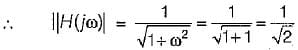and  ∠H(jω) = -tan-1ω = -tan-1(1)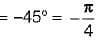∴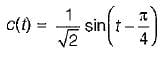Test: Frequency Domain Analysis of Control Systems- 3 - Question 8

A system has transfer function equal to (1-s)/(1+s). Its gain at ω = 1 rad/s is

Detailed Solution for Test: Frequency Domain Analysis of Control Systems- 3 - Question 8

Given transfer function represents an all pass filter which has gain of 1 at all frequencies.

Test: Frequency Domain Analysis of Control Systems- 3 - Question 9

The gain margin of a unity negative feedback system having forward path transfer function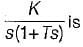Detailed Solution for Test: Frequency Domain Analysis of Control Systems- 3 - Question 9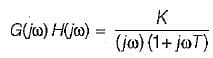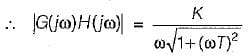∠G(jω) H(jω) = -90° - tan-1ωT = ϕ
At ω = ωpc,
ϕ = -180º
∴ -90° - tan-1ωpc = -180° or, ωpc = ∞ rad/s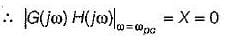∴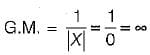Test: Frequency Domain Analysis of Control Systems- 3 - Question 10

The characteristic equation of a closed loop control system is given by s2 + 4s + 16 = 0. The resonant frequency (in radian/sec) of the system is

Detailed Solution for Test: Frequency Domain Analysis of Control Systems- 3 - Question 10

Given, s2 + 4s+ 16 = 0
or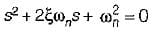and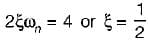∴ Resonant frequency,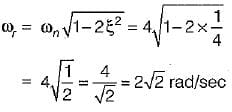Test: Frequency Domain Analysis of Control Systems- 3 - Question 11

The gain margin (in dB) of a system having the open-loop transfer function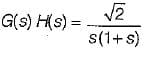is

Detailed Solution for Test: Frequency Domain Analysis of Control Systems- 3 - Question 11

Given,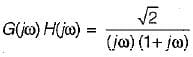∴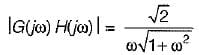and ∠G{jω)H(jω) = -90° - tan-1 ω = ϕ
At ω = ωpc, ϕ = -180°
∴ -90° - tan-1 ωpc = -180° or ωpc = ∞
∴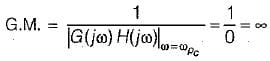Test: Frequency Domain Analysis of Control Systems- 3 - Question 12

Nichol’s chart is useful for detailed study and analysis of

Detailed Solution for Test: Frequency Domain Analysis of Control Systems- 3 - Question 12

Nichol’s chart consists of magnitude and phase angle of a closed loop system represented as a family of circle known as M and N-circle.

Test: Frequency Domain Analysis of Control Systems- 3 - Question 13

The forward path transfer function of an unity feedback system is given by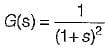What is the phase margin for this system?

Detailed Solution for Test: Frequency Domain Analysis of Control Systems- 3 - Question 13

Given,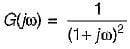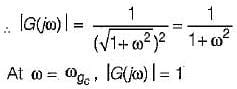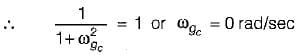Now,
∠G(jω) = -2tan-1ω = ϕ
At
ω = ωgc = 0 rad/s
ϕ = -2 x 0 = 0º
∴   P.M. = 180° + ϕ
= 180° + 0° = 180° = π

Test: Frequency Domain Analysis of Control Systems- 3 - Question 14

The closed loop system having the open loop transfer function,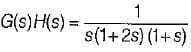is

Detailed Solution for Test: Frequency Domain Analysis of Control Systems- 3 - Question 14

Given,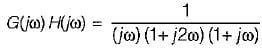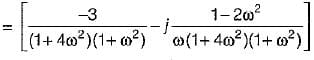For finding ωgc, Img[G(jω)H(jω)] = 0
or,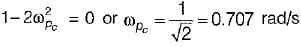Now,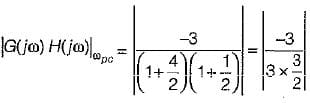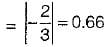Thus, Nyquist plot will cut real axis at -0.66.
The given system: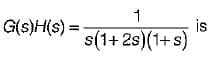of type-1 and order-3.
Therefore, Nyquist plot will be as shown below: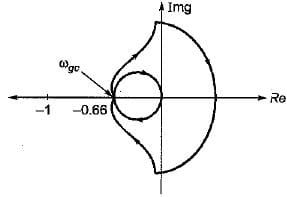Now, from above Nyquist plot,
N = 0 = Number of encirclements.
Also, P = number of open loop poles in RH s-plane = 0
Now, N = P-Z
or, 0 = 0 - Z or Z = 0
Thus, no. of closed loop poles in RH s-plane = 0.
Hence, system is stable.

Test: Frequency Domain Analysis of Control Systems- 3 - Question 15

The nyquist plot for a unity feedback control system having open loop transfer function G(s) = K(1-s)/(s+1) is shown in figure below :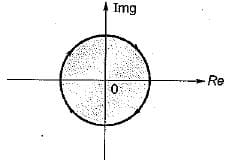The system is

Detailed Solution for Test: Frequency Domain Analysis of Control Systems- 3 - Question 15

Given,
G(s)H(s) = K(1-s)/(s+1)
or,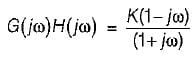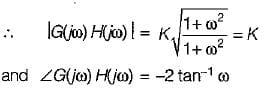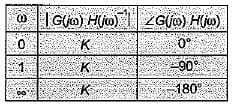Thus, the radius of Nyquist plot will be K.
Now, no.of open loop poles in RH s-piane = 0.
When K > 1, no. of encirclement, N = -1
When K< 1, no. of encirclement, N = 0
Thus, for K > 1, N = P - Z
or,    -1 = 0 - Z or Z = 1
∴ System is unstable.
For K < 1, N = P - Z or 0 = 0 - Z or Z = 0
∴ System is stable..

Test: Frequency Domain Analysis of Control Systems- 3 - Question 16

A unity feedback control system has a forward path transfer function of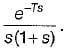Its phase value will be zero at a frequency of ω1.
Which one of the following equation should be satisfied for it?

Detailed Solution for Test: Frequency Domain Analysis of Control Systems- 3 - Question 16

Given,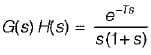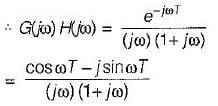or, ∠G(jω) H(jω) = -ωT - 90° - tan-1 ω
At ω = ω1, ∠G(jω) H(jω) = 0°
∴ -ω1 T-90° -tan-1ω1 = 0
or, tan-1ω= - (ω1T + 90°)
or, ω1, = -tan (90° + ω1T) = cot ω1T
∴ ω1 = cot(ω1T)

Test: Frequency Domain Analysis of Control Systems- 3 - Question 17

What is the approximate value of gain margin in the Nyquist diagram shown below?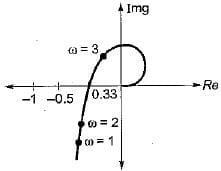Detailed Solution for Test: Frequency Domain Analysis of Control Systems- 3 - Question 17

At ω = ωgc,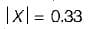(Using Nyquist diagram)
∴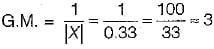Test: Frequency Domain Analysis of Control Systems- 3 - Question 18

The magnitude-frequency response of a control system is given in figure below: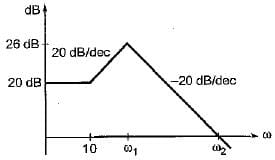The values of ω1 and ω2 are respectively

Detailed Solution for Test: Frequency Domain Analysis of Control Systems- 3 - Question 18

Number of decade change from first break frequency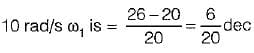Now,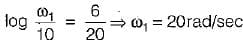Now, no. of decade change from break frequency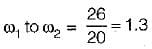∴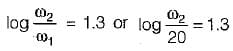or,

Test: Frequency Domain Analysis of Control Systems- 3 - Question 19

Match List-I with List-Il and select the correct answer using the codes given below the lists:
List-I
A. Resonant peak
D. Peak overshoot
List - II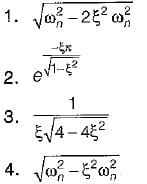Codes:
A B C D
(a) 3 4 1 2
(b) 3 1 4 2
(c) 2 4 1 3
(d) 2 1 4 3

Test: Frequency Domain Analysis of Control Systems- 3 - Question 20

A second order underdamped system has a damping ratio of 0.8. It is subjected to a sinusoidal input of unit amplitude. It has resonant peak of

Detailed Solution for Test: Frequency Domain Analysis of Control Systems- 3 - Question 20

Resonant frequency is given by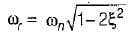For resonant peak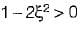or,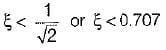Since ξ > 0.707, therefore there is no Mr (resonant peak).

## GATE ECE (Electronics) 2023 Mock Test Series

21 docs|263 tests
 Use Code STAYHOME200 and get INR 200 additional OFF Use Coupon Code
Information about Test: Frequency Domain Analysis of Control Systems- 3 Page
In this test you can find the Exam questions for Test: Frequency Domain Analysis of Control Systems- 3 solved & explained in the simplest way possible. Besides giving Questions and answers for Test: Frequency Domain Analysis of Control Systems- 3, EduRev gives you an ample number of Online tests for practice

## GATE ECE (Electronics) 2023 Mock Test Series

21 docs|263 tests